`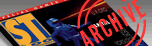ST-Log ISSUE 23 / SEPTEMBER 1988 / PAGE 64`

 C-MANSHIP BY CLAYTON WALNUM Here we are, back again after a month's respite (did you miss me?), and I think that this time around we'll tackle a subject that we've managed to avoid these last 2 1/2 years—the Atari ST's real-time clock. Actually, "avoid" probably isn't a good word to use here, since reading and setting the ST's clock is really not very hard. You just need to become proficient with handling data in a bitwise fashion rather than as words or bytes. And as we'll soon see, attaining those skills will not require an inordinate amount of effort, and what you'll learn will be a valuable addition to your future C programming projects. But first you should get this month's sample program up and running, and that involves a little more work than usual. You're going to need to create the dialog box shown in Figure 1. There are two ways you can do this. The first is to type in Listing 3 with ST BASIC (make sure you check your typing with ST Check), and then run it. The program will create the necessary resource file for you. The other way to produce the dialog box is to use a resource construction program to create it for yourself. It's a fairly simple dialog box, so this should be an easy task. The dialog contains only four objects—the editable text fields showing the time and date and the two exit buttons—but they must be created and named carefully. The dialog box itself is named DATEDIAL. The OK button is named OKBUTN and is simply a shadowed, exit button. The CANCEL button is named CANBUTN and is also a shadowed, exit button, but it is set as the default. The "Time" field is an unboxed, editable text string that is named TIMEFLD. Its ptmplt, pvalid and ptext strings, shown in order, are: ```Time:__:__:__ __ 999999AA 000000AM ``` The "Date" field is also an unboxed, editable text field. It's named DATEFLD, and its ptmplt, pvalid and ptext strings, also in their respective order, are: ```Date: __/__/__ 999999 000000 ``` That's all you need to know to reproduce the dialog box shown in Figure 1 (except that you must name the .RSC file DATE.RSC). If all of this sounds confusing to you, either review the C-manship columns on dialog boxes (Issues 13 and 14) or use Listing 3 to create your resource file. Now that you've created your resource file, you may type in Listing 1 and compile it. If you used the ST BASIC program to create your resource file, you must also type in Listing 2 before you try to compile the program. Save this listing to disk as DATE.H. Now run the program. If you've got the resource file in the same directory as the program, you'll see the dialog box shown in Figure 1. (If you're missing your resource file, the program will warn you, and then return to the Desktop.) The time and date shown in the dialog box are the current settings of your system clock. If you'd like to reset the clock, just edit the time and date strings and click on the OK button. If the strings you've entered are valid, the program will reset your system's clock and return to the Desktop. Otherwise you'll receive an error alert box, and you'll have to re-enter the information. If you're satisfied with the time as it is, click on the CANCEL button or simply press RETURN. Computer dating Let's take a look at Listing 2 and see what's going on here. You should already be familiar with most of what we're doing in this program. For instance, we long ago discussed how to load a resource file and get a dialog box up on the screen. In case you've gotten a little rusty over the last few months, the program listing is commented so that you should be able to see what's being done. Take a look at the function get__date(). It's here that we retrieve the system date from the computer's clock and convert it into a form that we can use in our dialog box. First we get the time with the call ```date = Tgetdate (); ``` where date is an integer. The function Tgetdate() is defined in your osbind.h file as gemdos(0×2a) and returns all the information we need to figure out the current date. Piece of cake, right? Not quite. If your noodle is active today, you'll remember that our dialog box displays the date by month, day and year. However, the Tgetdate() call yielded us only one value. See a problem here? To simplify the process of storing and passing the system date, the people who designed your ST's operating system decided to cram all the information we need to extract the current month, day and year into a single integer; and if you're really on the ball today, you'll real ize that that means we're going to have to finagle some bits to separate the information we want from the information we don't care about. The system time returned from the Tgetdate() function is formatted in the following manner. Bits 0 to 4 (counting from right to left, remember) contain the day, bits 5 to 8 contain the month, and bits 9 to 15 contain the year since 1980, or, in other words, the current year minus 1980. Figure 2 illustrates this format. What we have to do is figure out a way to extract the day, month and year from the entire integer. Thank heavens for bitwise operations! A bit about bits The C programming language supplies us with five operators to manipulate the bits that make up a piece of data. Some of them you've seen before; a couple of them are new to you. Those operators are: ```& Bitwise AND ^ Bitwise exclusive OR | Bitwise inclusive OR << Left shift >> Right shift ``` We've already had experience with the bitwise AND and bitwise inclusive OR operators. The AND operator compares the bits of two values and places into the result a 1 in any position where both bits of the compared values are set and a 0 in every other case. This allows us to "mask" out the bits in a value that we're not interested in. We create a mask by setting the bits of the mask that correspond to the bits we wish to extract from the value of interest. Every other bit is turned off. Let's say we wanted to get the value of the low byte of a word. We would create a mask that looked like this: Suppose the value we want to extract information from is called number and the binary value that number contains is 0010110101100110. The calculation would look like this: ```0010110101100110 number 0000000011111111 Mask ---------------- 0000000001100110 Result ``` As you can see, the result contains only the bit values we wanted to retain. In a C program the above calculation would be written as: ```result = number & 0 x 00ff ``` The inclusive OR operator is almost the opposite of the AND operator. Rather than extracting portions of a value, it lets us insert them. When you inclusive OR two values together, the result will have a bit set wherever there was a bit set in either one or both of the compared values. Let's say we wanted to merge the values contained in two variables called var1 and var2. The binary value contained in var1 is 0000000010101011, and the binary value contained in var2 is 1101101100000000. The inclusive OR operation looks like this: ```0000000010101011 num1 1101101100000000 num2 ---------------- 1101101110101011 Result ``` You can see from the result that we've combined the low byte of num1 with the high byte of num2. There's one important thing you must be aware of, though. This combining of values will work only when the positions that will hold the merged value all contain zeroes. In other words, we would not get the proper result in the above operation if the high byte of num1 was not cleared: ```1111111110101011 num1 1101101100000000 num2 ---------------- 1111111110101011 Result ``` The same problem would crop up if the low byte of num2 hadn't been cleared. A bitwise exclusive OR is similar except that the result will contain a 1 only in those positions where either one or the other bit is set. If both bits are set or both bits are cleared, the result will be a 0. The left-shift operator causes the bits in the first operand to be shifted to the left the number of times indicated by the second operand. The right-hand, emptied bits will be filled with zeroes. For instance, let's take a variable named num that contains the binary value 1011010110101101. If we were to perform the operation num<<5, the result would be 1011010110100000. The right-shift operator works much the same way, except that the emptied left-hand bits may or may not be zero-filled, depending on the machine and data type you're using. The rule is if the data type is unsigned, you are guaranteed to get a zero fill; otherwise, the left-hand bits may (depending on the machine) be filled with the value of the sign bit (the most significant bit). But what about the date? So here we are, finally back to the original problem of extracting the day, month and year from the single integer returned from the Tgetdate() call. Think about it for a minute. Have you got it figured out yet? No? Let me explain then. The information we need for the day is contained in bits 0 through 4, right? So, what we need to do is mask out bits 5 through 15. Then our result will contain only the value stored in the lower five bits—and that value is the current day. (Of course, whether or not this value matches your wall calendar depends on whether your system clock has been set properly.) Let's say the value returned from Tgetdate() is the one shown in Figure2. Figure 3a then illustrates the operations involved in extracting the day. We first create a mask we can AND with our integer—a mask that will ensure that bits 5 through 15 in our result will be clear, while at the same time maintaining the values of the lower five bits. The proper mask is 0000000000011111 in binary or 0x00If in hexadecimal. (Note that it's much easier to create your mask in binary first then convert it to hexadecimal. That way you can easily see which bits you're setting.) Then all we have to do is AND the system date with our mask. In Listing 1, the line that accomplishes this feat looks like this: ```day = date & 0x00lf; ``` Say! That was pretty easy, wasn't it. The next step is to get the month, but we run into a complication right away. If we were to just AND out the bits we weren't interested in, we'd end up with the value 0000000101100000 which translates to a decimal value of 352. Ouch! When's the last time you saw a month numbered 352 on your calendar? To get the value we really want, we have to move the four bits we're interested in to the right five places. Sounds to me like a good job for the right-shift operator to handle. But let's perform the shifting first and then mask out the unnecessary bits. That way we make sure we don't get any garbage in the upper bits as a result of the shift operation. Theoretically, it would work either way, since our sign bit will be a zero. But I learned a long time ago that, when it comes to computers, you can only trust what you're certain of. And I'm certain that if I do the AND operation last, I'll have the result I'm looking for. In Listing 1, the line that gets us our month looks like this: ```mnth = (date >> 5) & 0x000f; ``` These operations are illustrated in Figure 3b. Finally, to get the year, we have to do the same operation, only we'll be shifting the bits down nine places instead of five, and we'll be using a different mask because we're interested in a different number of bits. Figure 3c illustrates this operation, and the equivalent line in Listing 1 looks like this: ```year = ((date >> 9) & 0x007f) + 86; ``` Although Figure 3c doesn't show it, we have to remember to add 80 to the result because, as I mentioned before, the year returned from the Tgetdate() call is the year since 1980. Some timely information Now let's look at the function Get__time() in Listing 1. We get the system time with the call ```tine = Tgettime (); ``` where time is an integer. Bits 0 through 4 of this value will contain the seconds divided by two, bits 5 through 10 will contain the minutes, and bits 11 through 15 will contain the hour. We can extract this information in the same way we calculated the date—by shifting the bits we're interested in all the way to the right, and then using a mask and the AND operation to clear the bits we're not interested in. I don't think we need to go into a lot of detail here, but there is one thing I want to mention—something that we didn't have to deal with when we calculated the date. The value for the hour portion of the system time is in 24-hour format; that is, it'll be a value from 0 to 23. Values from 0 to 11 represent the hours of midnight to 11 a.m., and the values from 12 to 23 represent the hours from noon to 11 p.m. To make the time more readable to the user, our function get__time() does some converting so that the time will be displayed in the manner we're most used to seeing it. (Of course, if you're in the military, you may not approve of this conversion!) Also, keep in mind that the value for the seconds is the number of seconds divided by two. This means that you must multiply times two the value for the seconds returned by the Tgettime() function. This also means that your ST's clock is only accurate to the nearest even second. Setting the time and date Setting the system's time and date requires only that we reverse the process we used to get the time and date. Instead of using an AND operation, we'll be using the inclusive OR, and instead of shifting bits to the right, we'll be shifting them to the left. In Listing 1, the function set___date() handles both the setting of the time and the setting of the date. To set the time, we use the call ```Tsettime (time); ``` where the integer time uses the same bit format we studied when we discussed the Tgettime() call. To set the date, we use the call ```Tsetdate ( date ); ``` where the integer date uses the same bit format we learned about when we discussed the Tgetdate() call. These functions are defined in your osbind.h file. Let's take just a quick look at how we prepare the integers for these calls, and let's use time as our example this time around. Suppose the time we wanted to set the system clock to was 14:36:34 (that's 2:36 p.m. for those of you who could never get the hang of a 24-hour clock). Setting the seconds is easy: ```time = seconds; ``` Here, seconds is equal to 17. (Remember that the number of seconds must be divided by two; that's the only way the designers of the operating system could get the system time to fit into an integer.) Now time contains the binary value 0000000000010001, which equals 17 in decimal. Our value for minutes is 36, which is 0000000000100100 in binary. We have to move this information up into bits 5 through 10. The operation ```minutes=minutes<<5 ``` gives us a result of 0000010010000000 which is exactly what we want. Now we have to combine the seconds (the value of which is already stored in time) with the minutes. The operation ```time = time | minutes ``` does the trick handily. On |a binary level that operation looks like this: ```000000000010001 Seconds 000001001000000 Minutes ----------------- 0000010010010001 Time ``` To add the hours, we do the same sort of operation, only we'll be shifting the value for hours 11 places to the left. I might also add that it doesn't matter in what order we store the seconds, minutes and hours, as long as we follow the general procedure outlined above. If you look at Listing 1, you'll see that I started with the hours instead of the seconds. All ashore who's going ashore That about covers it. As you peruse this month's program, you may come across a couple of functions that aren't familiar to you. If so, just look them up in your manual. There's nothing complicated with any of them, and you should be easily able to figure out how everything in the sample program works. figure 1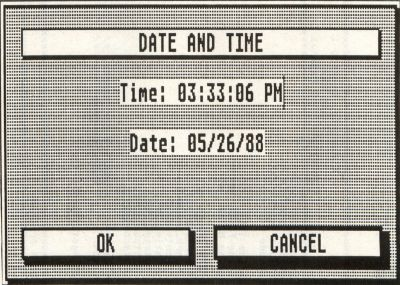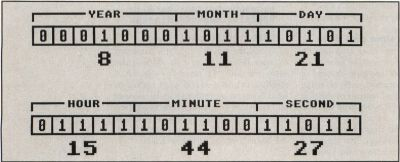figure 2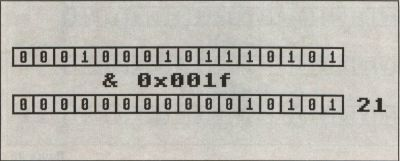figure 3a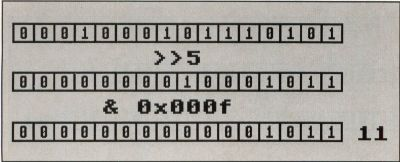figure 3b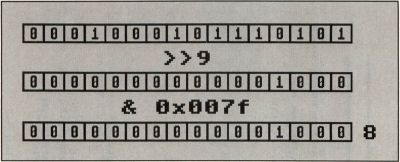figure 3c